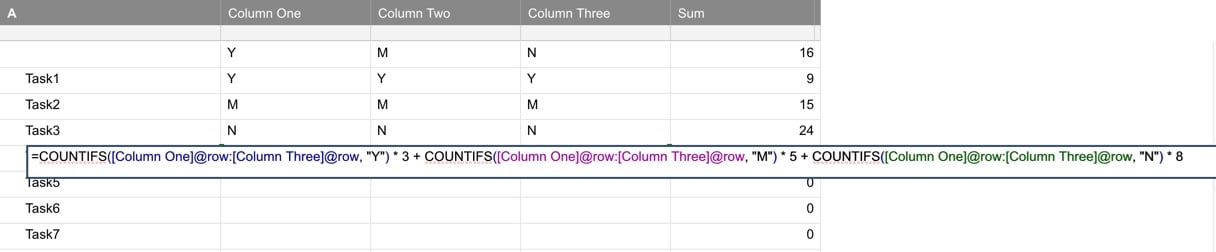# SUM more results from more IF conditions

Hi All,

image different questions (1 for each column) with 3 possible drop-down answers (Yes, Maybe, Not). Every combination question/answer generate a different score.

Ex: Question 1

• Y - 3 points
• M - 5 points
• N - 8 points

I need to have in a single cell the sum of the score of a gruop of question.

Have you any tips or suggestion for me?

Fabio

Tags:

• Hi Fabio,

Can you share some screenshots or other helpful information?

I hope this helps you!

Best,

Andrée Starå

Workflow Consultant @ Get Done Consulting

SMARTSHEET EXPERT CONSULTANT & PARTNER

Andrée Starå | Workflow Consultant / CEO @ WORK BOLD

W: www.workbold.com | E:[email protected] | P: +46 (0) - 72 - 510 99 35

Feel free to contact me for help with Smartsheet, integrations, general workflow advice, or anything else.

• It seems as if a simple SUM function should work for you once you designate the ranges to sum since you only want to sum a group regardless of the answer.

• =countif(A:A,"Y") * 3 + countif(A:A,"M") * 5 + countif(A:A,"N")*8

Where the column name is A you can have different columns or whatever, just change it to suit your needs. if you want a by row solution you need if statements

• @Luke. Seems like you could use your same formula but adjusted to shoot across the row. You would just need to assign the row number to the range and drag fill it down to calculate for each row. Assuming your column names were Column One, Column Two and Column There, you could use the following.

=COUNTIFS([Column One]@row:[Column Three]@row, "Y") * 3 + COUNTIFS([Column One]@row:[Column Three]@row, "M") * 5 + COUNTIFS([Column One]@row:[Column Three]@row, "N") * 8

This worked for me. You could have as many columns as you wanted, you just need to make sure that you list the first and last one in the series...• Dear All,

This is the correct formula for me:

=COUNTIFS([Column One]2; "Y") * 3 + COUNTIFS([Column One]2; "M") * 5 + COUNTIFS([Column One]2; "N") * 7 + COUNTIFS([Column Two]2; "Y") * 1 + COUNTIFS([Column Two]2; "M") * 4 + COUNTIFS([Column Two]2; "N") * 9 + COUNTIFS([Column Three]2; "Y") * 3 + COUNTIFS([Column Three]2; "M") * 3 + COUNTIFS([Column Three]2; "N") * 2

Infact for each question (or column) scores are different, so I need to list all the possibility in the formula and I can't use @row.

Thanck you again for the support!

All the best,

Fabio Calandri

• Gotcha, that makes sense. You have different point values per column. You could still use @row as it will pull the row number for you. @row just pulls the row number that your formula is in... So if you aren't putting the tally in the same row, then you're right. Otherwise, if your tally is in the same row @row can replace the 2 so its easier to copy and paste into other rows.

## Help Article Resources

Want to practice working with formulas directly in Smartsheet?

Check out the Formula Handbook template!